# How to convert ml to grams and why is it necessary?

So, in measurement, there are various systems and units. The very purpose of units is to understand the kind of thing and dimension that you are measuring. So, by looking at the unit, we can understand two things. First of all, the kind of thing we are measuring. Let us say you are using a 50 grams weight, so you must be measuring something very light, like fruit. But, the fact that the weight is 50 grams, also shows that you are measuring the weight of the substance. So, gram is a unit we use for measuring mass or weight. However, the SI unit for measuring these quantities. Moreover, in real life, these quantities do not exist in isolation. So, you might often need to convert one quantity to another. Therefore, you may need to convert ml to grams.So, as we have already seen, a gram is a unit of mass or weight. On the other hand, ml or milliliters is a unit of volume. However, ml is not the SI unit of volume again which is liters. Therefore, if you convert ml to grams, you are trying to find out the mass of something from the volume that it occupies. So, we will see how these two are connected and how you can convert them. However, before that, let us have a better understanding of grams themselves and the various ways you can convert them.

## Convert ml to grams formula

The formula that you need to convert ml to grams is very simple. However, you need to note a few things before knowing it. So, the density of any substance is the amount of matter or mass that any substance contains per unit volume of it. Therefore, density = total mass/ volume. Hence, if we reverse the equation, we get volume = total mass/ density of the substance. Now, let us say the mass is in grams and the density is in grams per ml. So, if you divide the two, you will get the volume and be able to convert ml from grams.

However, the units are very important here. So, it may happen, the density that you know is in kg/ liter, or grams/ liter. Or the mass is in kg while the density is in grams/ liter. Therefore, you have to convert the mass from kg into grams. On the other hand, you also have to convert the density from grams/ liter to grams/ ml.

When you have done this, all your units have reached uniformity. Therefore, you can now cancel the mass units to find out the volume. Similarly, you can also find out the mass. However, to find out the mass you need to multiply the volume with the density of the substance. So, in this way you can convert ml to grams and vice-versa.

However, there is another condition too. More than ml or liter, the most common unit of volume is a cubic meter or cubic centimeter. So, if you want to convert ml to grams, you have to convert cubic centimeters to ml first. However, this is quite simple. So, 1 centimeter cube is exactly 1 ml. Therefore, they are interchangeable. But this does not hold true for the other quantities.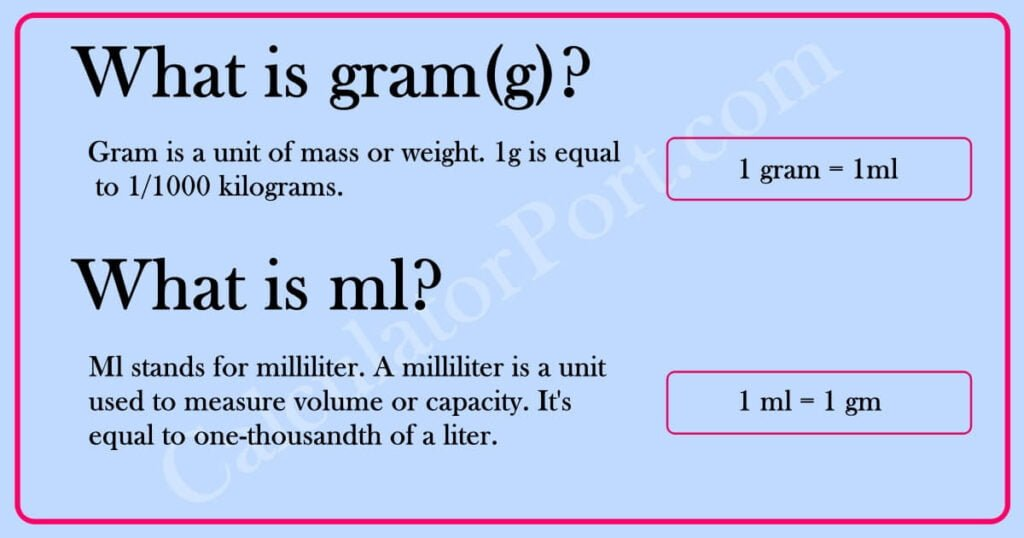## Convert ml to grams calculator

The process to convert ml to grams might get a little tricky sometimes. This is because you have to continuously ensure that all the concerned units are in uniformity. However, in times such as these, you have pretty much everything on the web. Therefore, you can refer to an online calculator that can convert ml to grams and vice-versa. So, you can use it if you either are unable to do the conversion or if you want to check the final value.

## Convert ml to grams using density calculator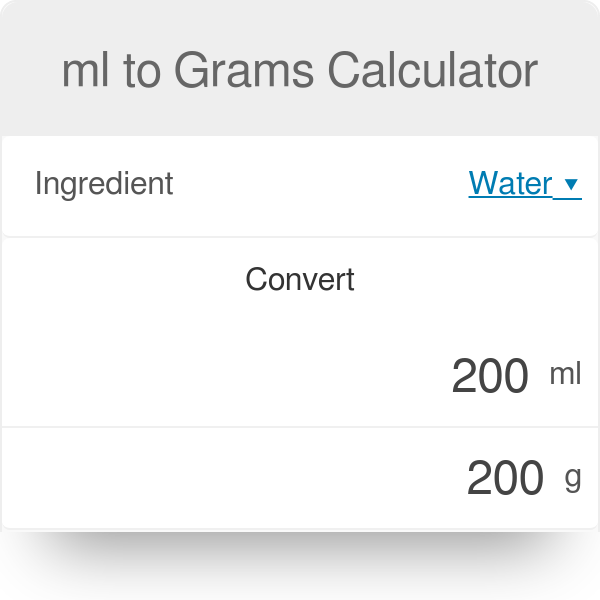We will see it working with the help of the one that Inch Calculator provides. Click on “Inch Calculator” to get the link that will guide you to the calculator.

## Step 1 to use an ml to grams calculator

So, on opening the calculator, you find two panels. On the first panel, the actual calculation where you want to convert ml to grams takes place. However, you need to study the second panel for here you find the process and the math behind the conversion. Once you understand it, your concepts will be clearer, and the chances of making mistakes will be lesser. Moreover, you can just do the conversion on your own now or verify the errors.

## Step 2 to use an ml to grams calculator

Now, in the first panel, you have to feed in 2 pieces of information. So, at first, you have a box that says “Ingredients” and a list appears from there. Therefore, you select the ingredient you are working on. It might be water, or granulated sugar, or anything in general. The calculator specifically asks for the material because each substance has a different density. Moreover, only water has 1 g/ml. Therefore, in the calculation, the density is important to convert ml to grams. Now in the box right below that also looks like a search engine and reads “Millimeter Value”, enter the value of the volume in millimeters.

So, for example, you choose the ingredient to be granulated sugar and type the millimeter value to be 3.

## Step 3 to use an ml to grams calculator

Now, click the “Convert” tab right below. So, in the box below, you find both the density of the substance that you have selected as well as your final answer, the conversion of ml into grams.

However, there is also a “Swap Units” tab. So, if you click it, you have to enter the value in grams and the calculator will convert it to ml. As for the rest, the process is exactly what you do to convert ml to grams.

## Convert ml to grams water

Now, as we probably all know water, the universal solvent has a density of unity. Therefore, water will have a density of 1 gram/ ml. Now, if the total mass of the water present is 1 gram. Therefore, the volume that water occupies will be 1 ml. So, whatever be the value of the mass in grams, for water, the value in ml will always be exactly the same.## Convert ml to grams oil

So, for cooking oil, the density is 0.88 grams/ ml. Therefore, when you convert ml to grams or vice-versa here, the value does not remain exactly the same as in water. So, 1 gram of cooking oil will occupy around 1.14 ml in volume.## Convert ml to grams sugar

So, powdered sugar again has a density of 0.56 grams/ ml. Therefore, when you convert ml to grams or the other way round, the two values will differ. 1 ml of powdered sugar will have a mass of 0.56 grams.## Convert ml to grams butter

So, butter is an oily emulsion. So, it must be quite dense. Therefore, butter has a density of 0.911 grams/ ml. Therefore, 1 gram of butter will occupy a volume of 1.097 ml. The two values again are different.## Convert ml to grams for ice-cream

Ice cream is quite a semi-solid, creamy substance. So, it has a density of 0.554 grams/ ml. Therefore, 1 gram of ice cream will be 1.805 ml in volume. So, again the volume is more than the mass when you convert ml to grams or vice-versa because the density is less than 1.## Convert ml to grams for gold

Now, this is a case that is very different from the previous ones. So, gold is a metal. It has a very high density, which is more than 1. So, gold has a density of 19.28 grams/ ml. Therefore, 19.28 grams of gold occupies 1 ml in volume. So, when you convert ml to grams, in this case, the value of the volume will be lesser than that of mass.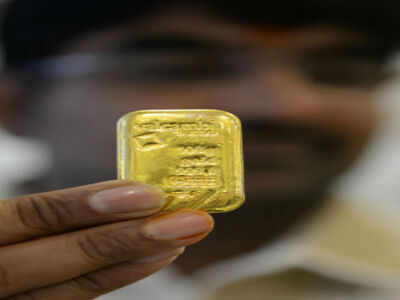## Convert ml to grams blood

So, blood is slightly more dense than water. It has a density of 1.12 grams/ ml. Therefore, 1.12 grams of blood occupy 1 ml in volume. Since the density is more than 1, like gold, the value of the volume will be lesser than that of mass.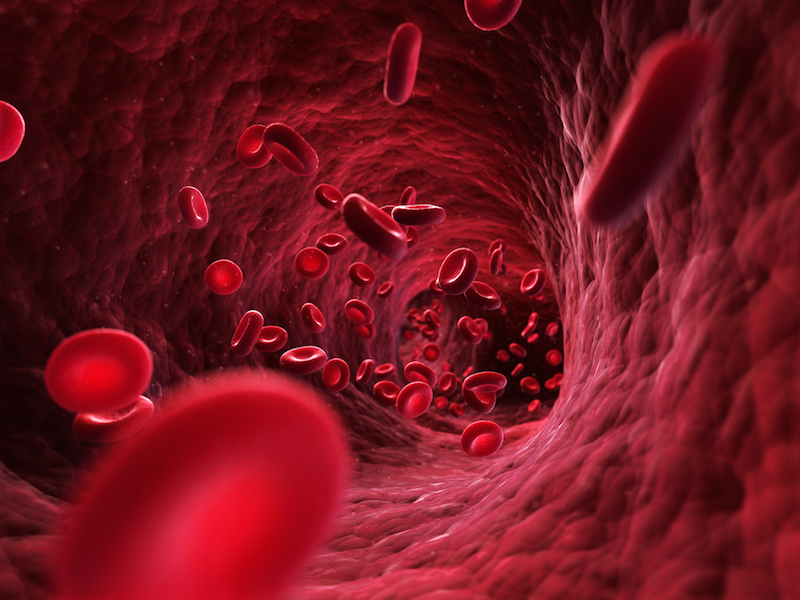## Grams to kg

So, kg or kilograms is the SI unit of mass. Now, initially, 1 kg was the mass of a solid cylinder made of platinum in the late 18th century. So, this rod was present in the International Bureau for weights and measures. However, scientists have changed the definition. This is because we need the definition of something more relative such that we can apply it easily in our daily lives. So, a kilogram was thought to be the same mass that 1000 cubic cm would occupy. However, scientists later found out that it was not exactly the same but something close by.

Now, you must know that a cubic cm is a unit of volume. Therefore, reaching liters and hence ml is quite easy from cubic cm. So, you can convert ml to grams and vice-versa even if you have the raw data either in kilograms or in cubic cm.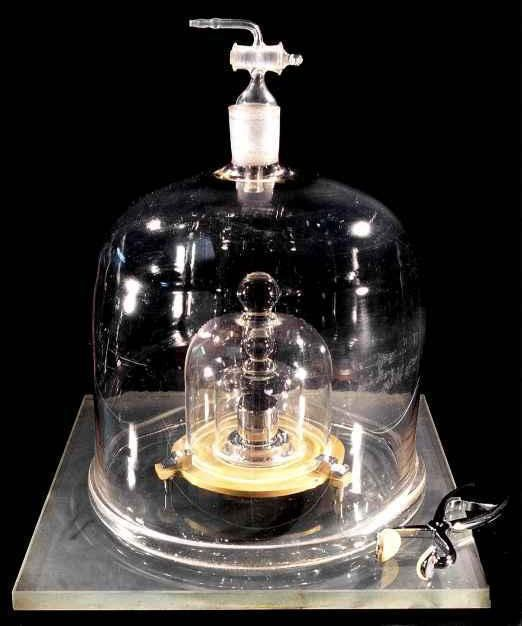Now, 1 kg means 1000 grams. So, as per the metric scale, between kilograms and grams, there are two different units- decagram and hectogram. Therefore, when you convert ml to grams, or vice-versa, make sure you have boiled down the mass unit to grams if the raw data is in kilograms. Moreover, you can simply do it by multiplying the kilograms coefficient by 1000. So, you will get the value of the mass of the substance in grams.

## Grams to mg

So, we have already seen that ml is a unit of volume. However, the word “milli” makes it very clear that it is a very small unit. Therefore, if you convert ml to grams, you must be handling something small, ideally. Similarly, mg must also be a very small quantity. Therefore, milligrams are one of the smallest quantities that we use to measure mass on a normal scale- that is to say not on the atomic scale.

Read Also: How many mL in a cup? The complete guide

So, one gram means 100 mg. Therefore, there are two places in between. They are decigram and centigram. Now, you might need to convert ml to grams or vice-versa but you have the data in mg. So, simply divide it by 100 to get the value of the mass in grams.

## Grams to atoms

Now, mass is not a quantity that we use only for bigger things- like to measure a vegetable or a bag. So, mass is an important part of almost every calculation that happens at the atomic level. This is because each invisible particle occupies some kind of space. However, they do not have mass in grams or mg. So, they need something more intricate like an atomic mass unit or amu. Hence, this is just an extremely small fraction of a gram, but how do you find it out?

So, in Chemistry, to find out the atomic mass unit, scientists divide the value in grams with Avogadro’s number. Now, this is 6. 02 x 10^23. So, this is the number of particles that one mole of a substance can contain. Therefore, to find grams from the atomic mass unit, you simply need to multiply the coefficient with Avogadro’s number.## Grams to moles

Now, moles are a concept that you find exclusively in chemistry. Therefore, this is not something that you will need in your everyday calculations. So, the number of moles of a substance is the ratio of the total mass of an element or compound in grams to the molar mass of the same substance. Therefore, you can understand that you cannot ever convert grams to moles or vice-versa. This is because grams are a proper unit of mass while the number of moles is practically a ratio. Rather, we can find the number of moles. Now, let us take a look at how exactly this works. However, before that, we need to know what molar mass is and how we can find it out.

So, to find out the molar mass of an element you just need to get hold of the mass of it from the periodic table. On the other hand, for a compound, you have to find out the masses of each of the individual elements, multiply them with the number of the atoms present and then add up the total thing. So, this is g/ mol. Now, in the numerator, place the total mass of the substance in grams. Therefore, on division, you cancel the grams, and what remains is the number of moles that you need right now.

## Convert ml to grams FAQs

### How many mls is in grams?

Ans. So, for unitary density, if you convert ml to grams or vice-versa, the value remains the same. Therefore, 1 ml means 1 gram.

### What is the difference between ml and grams?

Ans. So, ml or milliliters is a unit of volume. On the other hand, a gram is a unit that we use to measure the mass of a substance. The density of a substance connects these two units.

### Are 250 grams the same as 250 ml?

Ans. So, yes, 250 grams is the same as 250 ml but only when density is 1 gram/ mole. This means it holds true only for water. For every other ingredient, the density will change and so the two values will differ.

### Are milliliters bigger than liters?

Ans. No, milliliters are a much smaller unit than liters. One liter of a substance amounts to one thousand milliliters of the same substance.

### Is ml the same as gram flour?

Ans. So, the change of 1 gram unit in a gram flour measured 2.57 ml in volume as per the equivalent measure and for the same gram flour type.